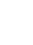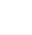﻿ Research & Development - Nanjing BKN Automation System Co.,LTD.NEWS

# Research & Development

Eddy current is one of the important features of fluid dynamics. In aerodynamics, eddy currents directly affect the flight characteristics of aircraft, and therefore must be considered in the design stage; in oceanography, the space-time evolution of eddy currents is an important means for scientists to explain ocean current circulation; in industrial fields, such as the entire internal combustion engine In the working cycle, complex and strong transient eddy currents are always carried out in the cylinder; therefore, eddy current testing is an important research topic, which belongs to the branch of the feature method in flow visualization. In addition, because the conductor induces a swirling current when it is moving in a changing magnetic field or relative to a magnetic field, eddy current testing is another research topic in the field of electromagnetism - far-field eddy current testing.

At present, eddy current testing standards at home and abroad can be roughly divided into three categories: point-based standards, geometric curve-based standards, and eddy current simulation methods. Point-based criteria usually assume that the eddy current is a region with a high rotational speed or that there is a point of minimum pressure as the vortex core, depending on the physical properties of the sample points within a single point or finite neighborhood in the eddy current, such as pressure, eddy current amplitude, and helicity. Calculate the physical properties such as pressure and velocity from the velocity vector or velocity gradient tensor of some sampling points; but the place with the least pressure is not necessarily where the vortex core exists, it may also be caused by other characteristics of the flow, common methods There are mainly low voltage methods, high spiral state methods, positive Q methods, eigenvector methods, and second-largest negative eigenvalue methods. This type of method can not extract the eddy current in all cases. The main problem is that the "weak" eddy current with less rotation speed and amplitude is not detected; the selection of sampling points has a great influence on the detection results; the probability of missed detection and false detection Higher; the first-order approximation also shows obvious defects, so an improved method of replacing the first-order differential with higher-order differential is proposed.

The geometry-based criteria are calculated using the geometric properties of the streamlines or veins, curvature, which is based on the intuitive concept of a spiral pattern around a set of central points, taking into account the geometry or kinematics of the flow. The feature above uses an instant streamline to represent the vortex. Typical methods are the curvature center detection method and the winding angle method. The curvature center detection method calculates the center of curvature of all the sampling points in the two-dimensional image. For the eddy current, the center of curvature of the surrounding streamline should be concentrated around a certain point, occupying a small grid, and thus the center point of curvature is performed. After meshing, it is sufficient to set a number of peak mesh regions with a large threshold search point density. This method has some similar shortcomings with the point-based detection standard. For example, the detection result is greatly affected by the sampling point selection, the missed detection and the false detection rate are high, etc., because the flow line is not a regular arc, the center of curvature.

The calculation also encounters many practical problems. The winding angle method selects the streamlines around a certain point to a cluster, and performs eddy current detection according to the bending angle and the distance setting standard. The advantage is that it overcomes the shortcomings of the point-based detection standard, can detect weak eddy currents with slower swirl speed and is easy to quantify calculation and evaluation. In this research work, Portela  gives the mathematical framework of eddy current testing in the two-dimensional case, corresponding to the spiral motion centered on a set of spatial points; and introduces the concept of differential geometry. The Jordan structure defines the geometric properties of the center point set and the wrap motion. In addition, although the eddy current region has Galilean invariance, most methods for extracting eddy current kernels rely on reference frames. Only the literature  detects the limitation of the eddy current core from the reference frame by extracting the ridge and valley lines of the eddy current region.

The methods described above are all based on the flow field characteristics expressed by mathematical methods for eddy current detection. In the presence of noise, they are often unstable, so some eddy current simulation methods appear: template matching method is used to calculate the flow field and design template. The maximum similarity value is used to extract the feature points of the flow field. This method reduces the mathematical calculation process and applies the convolution to the template matching of the flow field. The disadvantage is that it is sensitive to noise;

The continuous eddy current simulation method decomposes the flow into two parts: the eddy current and the quasi-Gaussian white noise of the organized structure by wavelet transform and then performs the eddy current extraction according to the threshold of the variance of the orthogonal wavelet coefficients at the multi-scale. The eddy current is extracted in. The detection is decomposed into two parts: eddy current kernel extraction and eddy current shell extraction. The former marks the velocity vector direction of the triangle mesh vertices based on Sperner lemma and finds the triangle mesh most likely to contain key points. The sub-method records the number and location of the intersections of the streamlines around the vortex shell, and at the same time plays the role of verifying the detection results of the former. The scale parameters in the method facilitate the development of an interactive eddy current detection method. The specific numerical simulation can be divided into three kinds: one is the Reynolds time-average method. Based on the Reynolds average motion equation and the pulsation equation, the assumptions are set according to theoretical analysis and practical experience to close the Reynolds average equation. A set of theoretical calculations for closed equations describing the mean turbulence. The most classical ones here are the kε− model and the algebraic stress model (ASM); direct numerical simulation (DNS) directly solves the eddy current motion through a very fine mesh. The three-dimensional unsteady Navier-Stoke equations calculate the instantaneous quantities in all scales and obtain the complete flow field information. The minimum scale vortices in the eddy current can be calculated. Although it can be accurately described, the huge storage and calculation amount are existing.

The computer is unbearable; the large eddy simulation method directly simulates the large-scale vortex in the eddy current by the instantaneous Reynolds equation, and the influence of the small vortex on the large eddy is considered by the approximate model, which is called the sub-grid stress scale model. Among these three methods, direct numerical simulation is the most complete method, with only two disadvantages: the calculation is huge and only applies to the lower Reynolds number; the Reynolds time-average method calculates the average value, the calculation amount is small, and the disadvantage is that the model has great influence and is only suitable for high Reynolds number. The large eddy simulation method is centered in all aspects. As a direct numerical simulation method, the large eddy simulation method can be used to obtain the velocity field of the main structure of the eddy current, which can reflect the eddy current more accurately. Continuous movement and development.

Among the above three types of methods, the first two types belong to the methods developed at different stages of development. Among them, point-based detection belongs to the early traditional methods, and the standard based on geometric curves is proposed based on the advancement of flow visualization technology. The eddy current simulation method is a method developed in different application fields and is closely combined with specific applications. Although some progress has been made in eddy current testing, there is currently no uniform definition of eddy currents, which brings great difficulties to research work. In this respect, only Roth Martin has compared and analyzed the definition of eddy currents in the existing research literature but still does not give a unified definition. Therefore, there are still some basic theoretical problems to be solved in eddy current testing. In addition, the winding angle method is a promising method in the geometric curve-based detection standard.

There are many aspects worthy of research on the improvement and expansion of this method, such as:

1) Integrating the key points on the streamline into the winding angle In the law, only the streamlines near the key points are tracked to avoid global search;

2) the method is extended to the three-dimensional space, but the curve needs to be expanded into a surface. There are many problems to be solved, which is not easy.

3) Ellipse detection, spatial domain matching and time-domain tracking are the techniques often used in eddy current testing. How to improve and improve these technologies in combination with practical applications also requires further research.

•3-102，No.1 Hengyi Road，Qixia District, Nanjing City, Jiangsu Province, China.
•Email Us
liuweifeng@bknzdh.com
•Call us on:
+86 18705164588